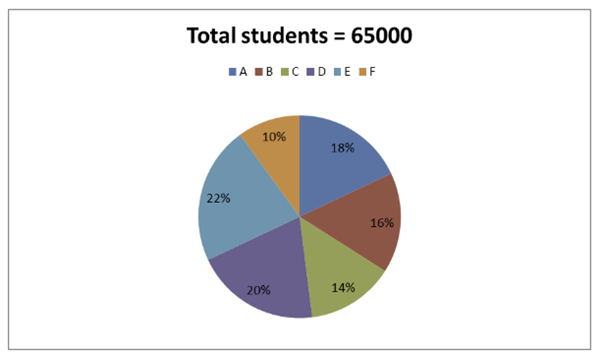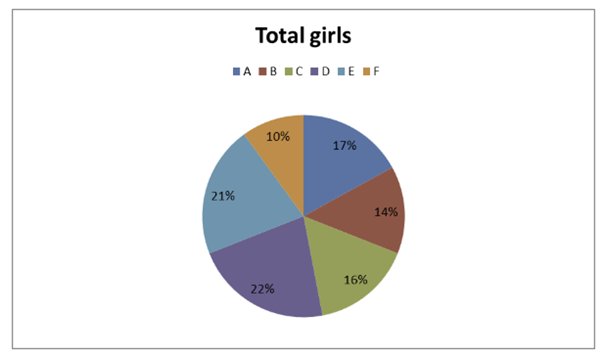# SBI Clerk Mains Quantitative Aptitude (Day-42)

Dear Aspirants, Our IBPS Guide team is providing new series of Quantitative Aptitude Questions for SBI Clerk Mains 2020 so the aspirants can practice it on a daily basis. These questions are framed by our skilled experts after understanding your needs thoroughly. Aspirants can practice these new series questions daily to familiarize with the exact exam pattern and make your preparation effective.

Start Quiz

Directions (01 – 05): Study the following information carefully and answer the questions given below.

The following pie chart1 shows the percentage distribution of total number of students appeared for the entrance examination from 6 different cities and the pie chart2 shows the percentage distribution of total number of girls appeared for the entrance examination from those cities. The ratio between the total number of boys to that of girls is 7: 6.1) Find the difference between the total numbers of boys appeared for the entrance examination from city A and E together to that from city B and D together?

A) 2000

B) 2400

C) 2600

D) 2800

E) None of these

2) If 60 % of boys from city C and 65 % girls from city F cleared their entrance examination, then find the sum of the total number of boys cleared their entrance examination from city C and total number of girls cleared their entrance examination from city F?

A) 4920

B) 4650

C) 4840

D) 4530

E) None of these

3) Find the difference between the average number of boys appeared for the entrance examination from city A, D and F and together the average number of girls appeared for the entrance examination from city A, C and E together?

A) 150

B) 100

C) 200

D) 250

E) None of these

4) If the ratio between the total number of girls cleared the entrance examination and went to the abroad for higher education to that of not cleared the entrance examination from city B and city D is 2 : 5 and 4 : 7 respectively, then find the total number of girls cleared the entrance examination and went to the abroad for higher education from city B and city D together?

A) 3200

B) 3000

C) 2800

D) 4000

E) None of these

5) If the percentage of total number of boys cleared the entrance examination and studied in IITs from city B and city E is 40 % and 35 % respectively, then find the ratio between the total number of boys cleared the entrance examination and studied in IITs from city B to that of total number of boys not cleared the entrance examination from city E?

A) 42 : 75

B) 28 : 47

C) 31 : 65

D) 55 : 79

E) None of these

Directions (06 – 10): The questions below are based on the given Series-I. The series-I satisfy a certain pattern, follow the same pattern in Series-II and answer the questions given below.

6) I) 4, 20, 60, 300, 900, 4500, 13500

II) 11 …… 2475. If 2475 is nthterm, then what value should come in place of (n + 2)thterm?

A) 34250

B) 18975

C) 12375

D) 37125

E) 25650

7) I) 924, 924, 462, 154, 38.5, 7.7

II) 534 ……. 4.45. If 4.45 is nth term, then what value should come in place of (n – 2)th term?

A) 89

B) 22.25

C) 72

D) 64.75

E) 45

8) I) 27, 1357, 2356, 3084, 3595, 3937

II) 55 ….. 4304. If 4304 is nth term, the find the value of n?

A) 7

B) 6

C) 8

D) 9

E) 5

9) I) 57, 66, 39, 84, 21, 102, 3

II) 72 ……. 36. If 36 is nthterm, then what value should come in place of (n+3)rdterm?

A) 117

B) 135

C) 18

D) 92

E) 53

10) I) 12, 24, 96, 576, 4608, 46080

II) 4 …… 184320. If 184320 is nthterm, then find the value of n?

A) 5

B) 6

C) 9

D) 7

E) 8

Directions (1-5) :

The total number of boys appeared for the entrance examination from city A and E together

= > [65000 * (18/100) – 30000 * (17/100)] + [65000 * (22/100) – 30000 * (21/100)]

= > [11700 – 5100] + [14300 – 6300]

= > 6600 + 8000 = 14600

The total number of boys appeared for the entrance examination from city B and D together

= > [65000 * (16/100) – 30000 * (14/100)] + [65000 * (20/100) – 30000 * (22/100)]

= > [10400 – 4200] + [13000 – 6600]

= > 6200 + 6400 = 12600

Required difference = 14600 – 12600 = 2000

The total number of boys cleared their entrance examination from city C

= > [65000 * (14/100) – 30000 * (16/100)] * (60/100)

= > [9100 – 4800] * (3/5)

= > 2580

The total number of girls cleared their entrance examination from city F

= > 30000 * (10/100) * (65/100)

= > 1950

Required Sum = 2580 + 1950 = 4530

The average number of boys appeared for the entrance examination from city A, D and F together

= > {[65000 * (18/100) – 30000 * (17/100)] + [65000 * (20/100) – 30000 * (22/100)] + [65000 * (10/100) – 30000 * (10/100)]} / 3

= > {[11700 – 5100] + [13000 – 6600] + [6500 – 3000]} / 3

= > (6600 + 6400 + 3500) / 3

= > 5500

The average number of girls appeared for the entrance examination from city A, C and E together

= > 30000 * [(17 + 16 + 21) / 100] * (1/3)

= > 5400

Required difference = 5500 – 5400 = 100

The total number of girls cleared the entrance examination and went to the abroad for higher education from city B and city D together

= > 30000 * (14/100) * (2/7) + 30000 * (22/100) * (4/11)

= > 1200 + 2400 = 3600

The total number of boys cleared the entrance examination and studied in IITs from city B

= > [65000 * (16/100) – 30000 * (14/100)] * (40/100)

= > (10400 – 4200) * (2/5)

= > 2480

The total number of boys not cleared the entrance examination from city E

= > [65000 * (22/100) – 30000 * (21/100)] * (65/100)

= > (14300 – 6300) * (13/20)

= > 5200

Required ratio = 2480 : 5200 = 31 : 65

Directions (6-10) :

Series I pattern:

4*5 = 20

20*3 = 60

60*5 = 300

300*3 = 900

900*5 = 4500

4500*3 = 13500

Series II pattern:

11*5 = 55

55*3 = 165

165*5 = 825

825*3 = 2475 (nth) term

2475*5 = 12375 (n + 1)th term

12375*3 = 37125 (n + 2)th term

Series I pattern:

924 ÷ 1 = 924

924 ÷ 2 = 462

462 ÷ 3 = 154

154 ÷ 4 = 38.5

38.5 ÷ 5 = 7.7

Series II pattern:

534 ÷ 1 = 534

534 ÷ 2 = 267

267 ÷ 3 = 89 (n – 2)th term

89 ÷ 4 = 22.25 (n – 1)th term

22.25 ÷ 5 = 4.45 (nth) term

Series I pattern:

27 + (113 – 1) = 1357

1357 + (103 – 1) = 2356

2356 + (93 – 1) = 3084

3084 + (83 – 1) = 3595

3595 + (73 – 1) = 3937

Series II pattern:

55 is first term.

55 + (113 – 1) = 1385

1385 + (103 – 1) = 2384

2384 + (93 – 1) = 3112

3112 + (83 – 1) = 3623

3623 + (73 – 1) = 3965

3965 + (63 – 1) = 4180

4180 + (53 – 1) = 4304

4304 is 8th term.

So, n = 8

Series I pattern:

57 + (9*1) = 66

66 – (9*3) = 39

39 + (9*5) = 84

84 – (9*7) = 21

21 + (9*9) = 102

102 – (9*11) = 3

Series II pattern:

72 + (9*1) = 81

81 – (9*3) = 54

54 + (9*5) = 99

99 – (9*7) = 36 (nth term)

36 + (9*9) = 117 (n + 1)th term

117 – (9*11) = 18 (n + 2)th term

18 + (9*13) = 135 (n + 3)rd term

Series I pattern:

12*2 = 24

24*4 = 96

96*6 = 576

576*8 = 4608

4608*10 = 46080

Series II pattern:

4 is the first term

4*2 = 8

8*4 = 32

32*6 = 192

192*8 = 1536

1536*10 = 15360

15360*12 = 184320

184320 is 7th term. So, n = 7

 Check Here to View SBI Clerk Mains 2020 Quantitative Aptitude Questions Day 41 Day 40 Day 39 Click Here for SBI Clerk 2020 – Detailed Exam Notification